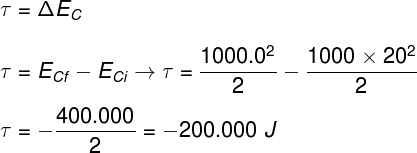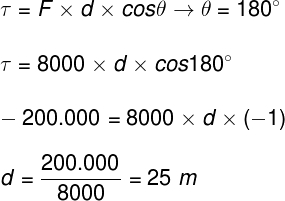# Dissipative forces

Dissipative forces are those capable of irreversibly transforming mechanical energy into less useful forms of energy, such as thermal energy. Friction and drag forces are common examples of dissipative forces, which are present in virtually all physical systems, such as machines and means of transport. The study of dissipative forces is part of Newton’s laws , in addition to being of great importance for some specific areas of dynamics , such as aerodynamics, fluid dynamics , etc.

## What are dissipative forces?

Dissipative forces are those that cause “losses” of mechanical energy in non-conservative systems, that is, those in which the total mechanical energy decreases as a function of time. Friction forces and drag forces are good examples.

When these forces act on a moving body, part of its kinetic and potential energy is converted into thermal energy , for example. Furthermore, the energy that is dissipated by this type of force cannot be spontaneously converted into mechanical energy, that is, this energy cannot be used after it has been dissipated. In other words, dissipative forces are not capable of generating potential energy, as conservative forces do.

## Conservative forces and dissipative forces

Conservative forces and dissipative forces differ in that the former, when they do work on a body, are able to endow it with potential energy , which is a form of energy that can be converted back into kinetic energy .

Furthermore, regardless of its nature, all potential energy is related to the different positions occupied by a body. Thus, it does not depend on the path along which a body or particle moved under the action of a conservative force, but only on its final and initial positions.

An example of the action of a conservative force is when, in a vacuum region , a ball is dropped at a certain height, so that it falls in free fall towards the ground, and then the experiment is repeated, but allowing the ball slides down a perfectly smooth inclined plane In these two cases, the kinetic and gravitational potential energies have equal magnitudes , for any equal instants of time and any equal heights, regardless of the trajectory taken by the ball. Therefore, it can be said that the gravitational force, or weight force, has a conservative nature.

On the other hand, analyzing the dissipative forces that could act on the described system, the situation would be completely different. Freely falling in a straight line, the ball could suffer the action of drag forces caused by its movement in the air, which depend on factors such as shape, mass, initial speed, etc. Rolling on the inclined plane, the ball would also suffer mostly the action of a dynamic friction force , which depends, among other factors, on the compression that the ball makes on the plane, as well as on the angle formed between the plane and the horizontal direction, for example.

## Example on dissipative forces

Let’s solve an example situation that illustrates the operation of a non-conservative system. In this system there is action of a friction force.

Example: A driver is driving a car with a speed of 20 m/s when he sees a red light. At the instant he slams on the brakes, the driver is 30 m away from the traffic light. Knowing that the brake exerts a frictional force of 8000 N on the car’s tires, determine how much space it takes for the car to come to a complete stop. Also, he answers: will the car be able to come to a complete stop before passing the traffic light?

Resolution:

First, let’s calculate what is the change in kinetic energy of the vehicle . Since this change is equivalent to the total work done by the friction force, according to the work and kinetic energy theorem , we will do the following calculation:Based on the result obtained above and with the formula used to calculate the work, we simply do the following:As the car travels 25 m until it comes to a complete stop, and the traffic light is 30 m after the start of braking, the vehicle will not pass the red light.

Check Also
Close